[BACK]Computer Modeling in Engineering & SciencesDOI: 10.32604/cmes.2021.015629

ARTICLE

Steganalysis of Low Embedding Rate CNV-QIM in Speech

Mechanical and Electrical Engineering College, Gansu Agricultural University, Lanzhou, 730070, China
*Corresponding Author: Wanxia Yang. Email: yanwanxia@163.com
Received: 31 December 2020; Accepted: 27 April 2021

Abstract: To address the difficulty of detecting low embedding rate and high-concealment CNV-QIM (complementary neighbor vertices-quantization index modulation) steganography in low bit-rate speech codec, the code-word correlation model based on a BiLSTM (bi-directional long short-term memory) neural network is built to obtain the correlation features of the LPC codewords in speech codec in this paper. Then, softmax is used to classify and effectively detect low embedding rate CNV-QIM steganography in VoIP streams. The experimental results show that for speech steganography of short samples with low embedding rate, the BiLSTM method in this paper has a superior detection accuracy than state-of-the-art methods of the RNN-SM (recurrent neural network-steganalysis model) and SS-QCCN (simplest strong quantization codeword correlation network). At an embedding rate of 20% and a duration of 3 s, the detection accuracy of BiLSTM method reaches 75.7%, which is higher than that of RNN-SM by 11.7%. Furthermore, the average testing time of samples (100% embedding) is 0.3 s, which shows that the method can realize real-time steganography detection of VoIP streams.

Keywords: CNV-QIM; steganography; BiLSTM; steganalysis; VoIP; speech

1  Introduction

Steganography is an important information security technology that not only hides the content of private information but also its existence to ensure secure transmission . Thus steganography technologies are highly valued by international academic community and military and other security sectors in many countries. As a countermeasure of steganography, steganalysis which is used to analyze whether secret information is hidden in the carrier is also a popular research topic, especially with the emergence of sufficient redundancy digital carriers .

The implementation of steganography relies on the carrier. The carriers of steganography could be any kind of data streams, such as images, texts, and audio. In recent years, Voice-over IP (VoIP) streaming media has grown rapidly, and it is difficult to identify due to real-time transmission via the Internet, which is very helpful for hiding secret information. Its massive payloads provide large information hiding capacity. Its multi-dimensional steganography makes detection very difficult. So VoIP becomes one of the best carriers of information hiding.

To reduce band width and improve real-time communication, however, low bit-rate speech codec, such as G.729 and G.723 coders, are used for data compression in VoIP transmission . Among the various compression methods, vector quantization (VQ) is the most popular technology in low bit-rate speech coding due to its high compression efficiency and ability to ensure superb voice quality, which also provides a good opportunity for hiding information. Because secret information can be hidden when optimizing the quantized codebook by quantization index modulation (QIM), whose action cannot damage the structure of the carrier with high concealment. Furthermore, the synthesis-by-analysis (ABS) framework applied in low bit-rate speech coding can effectively compensate for the additional distortion caused by the information hidden during residual quantization. These factors make steganalysis for QIM challenging. At present, the complementary neighbor vertices (CNV) algorithm based on graph theory proposed by Xiao et al.  is one of state-of-the-art VQ methods for hiding information, and it is the focus of this paper due to its detection difficulty.

Implementation process of the detection method in this paper is illustrated in Fig. 1. Its main characteristics are real-time and fast, the reason is that VoIP is dynamic and real-time, so the steganography detection in VoIP is also real-time, such as the real-time data acquisition. Secondly, if detection is performed offline, it is impractical to cache huge the VoIP data on the network. However, online real-time detection is available. Therefore, a sliding window with window length l and step s is designed to facilitate rapid testing online detection.Figure 1: Steganalysis process

The remainder of this paper is organized as follows. In Section 2, we discuss the research status of speech steganalysis. In Section 3, a new steganalysis method based on the BiLSTM model is proposed. In Section 4, the detection algorithms designed in Section 3 are simulated, and the experimental results are analyzed. Section 5 concludes our work.

2  Related Work

There are many successful methods for detecting steganography in speech coding, especially for LSB steganography. However, in terms of theoretical basis, there are two main categories of features used to steganalysis: the decorrelation of the Mel Frequency Cepstral Coefficient (MFCC) based on the human auditory principle, and the Linear Prediction Coefficient (LPC) and Cepstral Coefficient (LPCC) based on the human vocal mechanism. The former feature is mainly used in LSB steganalysis in high-speed speech coding with low compression rate, while the latter feature in low bit-rate parameter encoder is unsuitable for statistical analysis algorithm. The reason is that the meaning of each parameter in low bit-rate speech codec is not the same. Modifying some parameters by steganography will greatly reduce speech quality and even cause the distortion of speech signal. So the steganography in low bit-rate speech codec is usually implemented when optimizing codebook grouping, and the detection for it is totally different from that of LSB steganography. However, through the analysis of various steganography algorithms used in low bit-rate speech coding, corresponding steganalysis methods are proposed. For example, in , histogram flatness, characteristic functions and variance were applied to successfully detect the steganography of the fixed codebook index in the spatial domain. Based on [6,7] added the local extremum of the histogram and a 0, 1 distribution probability difference to improve detection performance. Reference  applied Markov correlation of the pulse bit to detect fixed codebook index steganography with a low embedding rate. By introducing the intra-frame and inter-frame codeword correlation network model, Li et al.  obtained accurate detection for pitch modulation steganography with certain detection difficulty in low bit-rate speech coding. References  extracted features of the distribution unbalance and loss correlation of codewords caused by steganography and achieved good detection results of QIM steganography of LPC in low bit-rate coders (G.723.1 and G.729). References  used multiple convolutional network to extract correlation features of the inputted signal to classify and achieved good results, but they lay particular emphasis on the analysis of steganographic speech at different duration.

For the above-mentioned methods, however, the features are manually selected and extracted for steganalysis, which has the following shortcomings. First, the manual selection of features cannot be applied well to the new steganography algorithm; i.e., it is not suitable for discontinuous CNV steganography. Second, voice data is a data stream for which the codewords of successive frames are correlated. However, traditional feature extract algorithms used small samples for classification cannot fully delineate the temporal information between the codeword sequence. Thus, how to fully mine information in the time and space domains of mass samples to improve the accuracy of detection is the crucial problem addressed in this paper. So the recurrent neural network (RNN) model with time memory is chosen to detect CNV-QIM steganography in low bit-rate speech codec in the paper, the model is further explained in the next subsection.

3  Speech Steganalysis Based on BiLSTM

3.1 BiLSTM Model

The LSTM model, which was proposed by Graves et al. in 2005 , solved the vanishing gradient problem by using gates to selectively memorize information. Thus, it has been successfully applied in speech recognition, natural language processing, etc. . For example, Lin et al.  used LSTM of RNN to obtain high-accuracy detection for QIM steganography in low bit-rate speech codec (G.729). However, language features, such as the causality between sentences, are logical. The standard LSTM model emphasizes the context information of past language while neglecting the context information of future language. To comprehensively express of semantic relationship between coding parameters in low bit-rate speech and the complete context information of language, the bidirectional LSTM network is designed to descript code-word correlation to get richer and more logical semantic features of speech. Fig. 2 shows a bi-directional RNN unfolded along the time axis.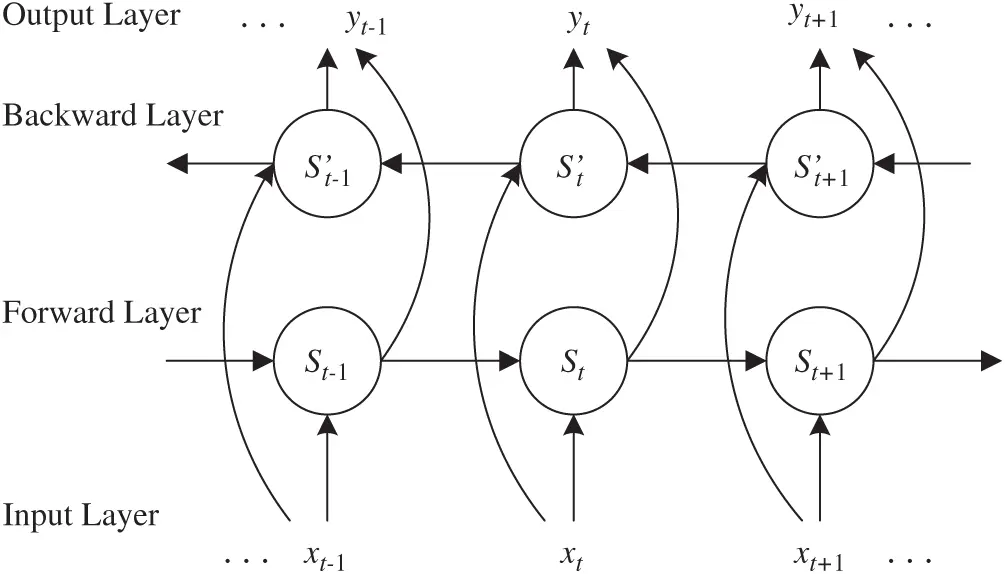Figure 2: BiLSTM model unfolding along the time axis

The composition output of two layers is as follows :

y=Wsyst+Wsyst(1)

It is obvious that the BiLSTM neural network can better express input information of model compared with LSTM, because it can learn and express the semantic information in speech of both the forward and backward.

3.2 Code-Word Correlation Model

Because speech are short-term stationary signals, they are segmented into short time frames in linear prediction coding, and each frame (10 ms for one frame in G.729 encoder and 30 ms for one frame in G.723 encoder) is analyzed to obtain the optimal LPC, which is then converted to a linear spectral frequency (LSF) quantized by three splitting vectors (s1, s2, s3). Here, each si (i=1,2,3) corresponds to a codebook Li (i=1,2,3), whose codeword space is{vi1,vi2,vi|Li|}, where vik represents the k codeword of the codebook Li and |Li| is the number of codewords in Li. Here, the codewords are one of the main parameters which contain rich voice information. Therefore, there is a strong correlation between the intra-frame and inter-frame codeword and is the main object analyzed in this paper. Which is easy to understand, because speech is the expression of language in the sound. Language is composed of words, and there are rich semantic relations and correlated between the forward and backward words. So the codewords in the coding streams of speech are correlative. In addition,  used the first-order Markov to model the codeword sequence of speech frame, and applied the state transition probability to quantify the correlation between codewords. However, QIM steganography will alter the correlation of the original codeword distribution while without changing the size of the codeword itself. In this paper, the distribution of the codeword in voice fragments of Chinese male and female is analyzed and the results are shown in Figs. 3a and 3b, respectively. It can be seen that the distribution significant change of the codeword before and after steganography in the experiment. So we design a model based on BiLSTM to delineate distribution change of codeword in codebook Li caused by steganagraphy. We then use softmax to distinguish cover speech and stego speech. The specific steps of establishing the model are as follows: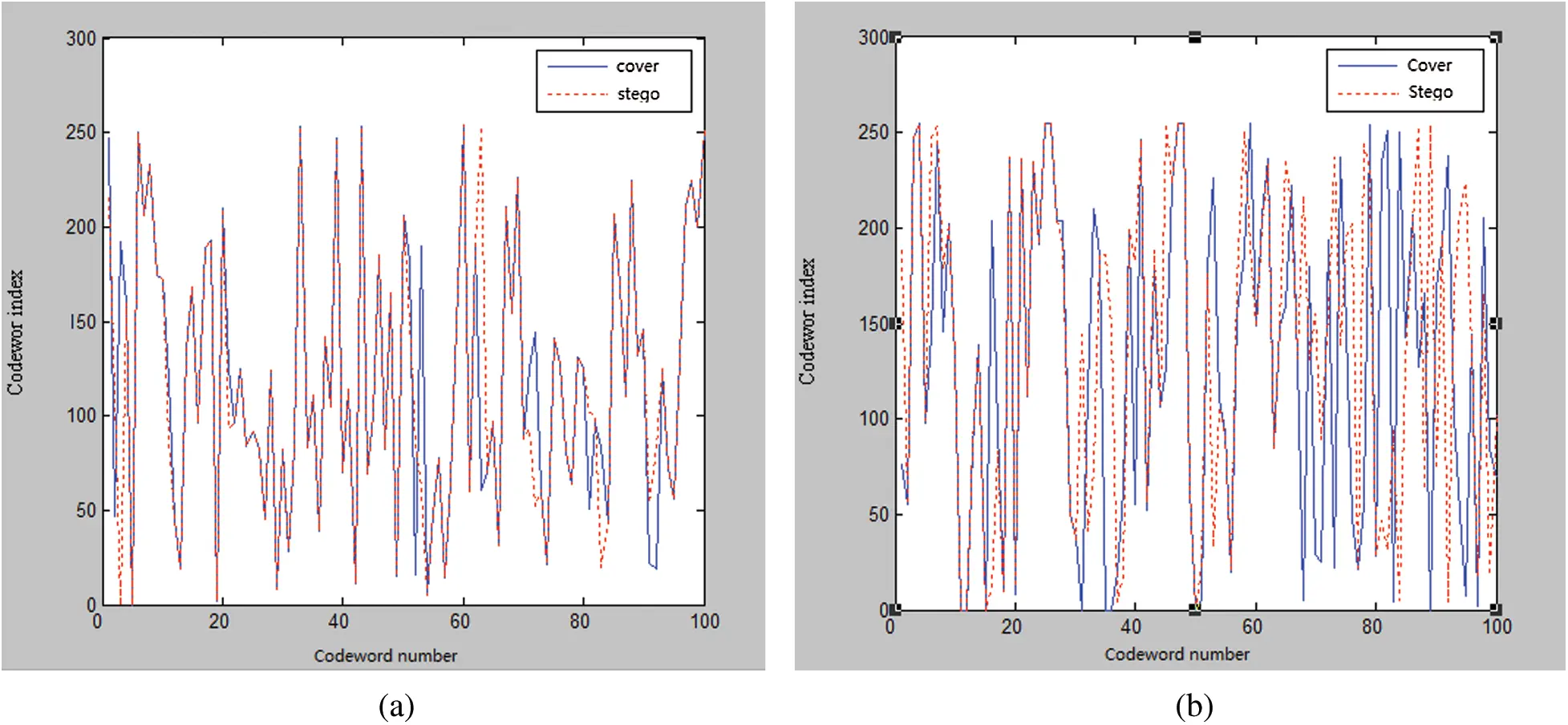Figure 3: Disturbance to the codeword sequence by the CNV-QIM steganography (a) male (b) female

The model constructed in this paper consists of two layers of LSTM units (as in Fig. 4). The first layer is the input layer with N1 BiLSTM units defined as U1={u1,1,u1,2u1,n1}, and the second layer contains N2 standard LSTM units defined as U2={u2,1,u2,2u2,n2}, where N1 and N2 are both 50 determined by experiment in case of considering detection accuracy and efficiency. If the conversion function of the LSTM unit is defined as f, then when the input sequence is I={i1,i2it}, the output sequence is O={o1,o2ot}, as follows:

Oj=f(I1:j)(2)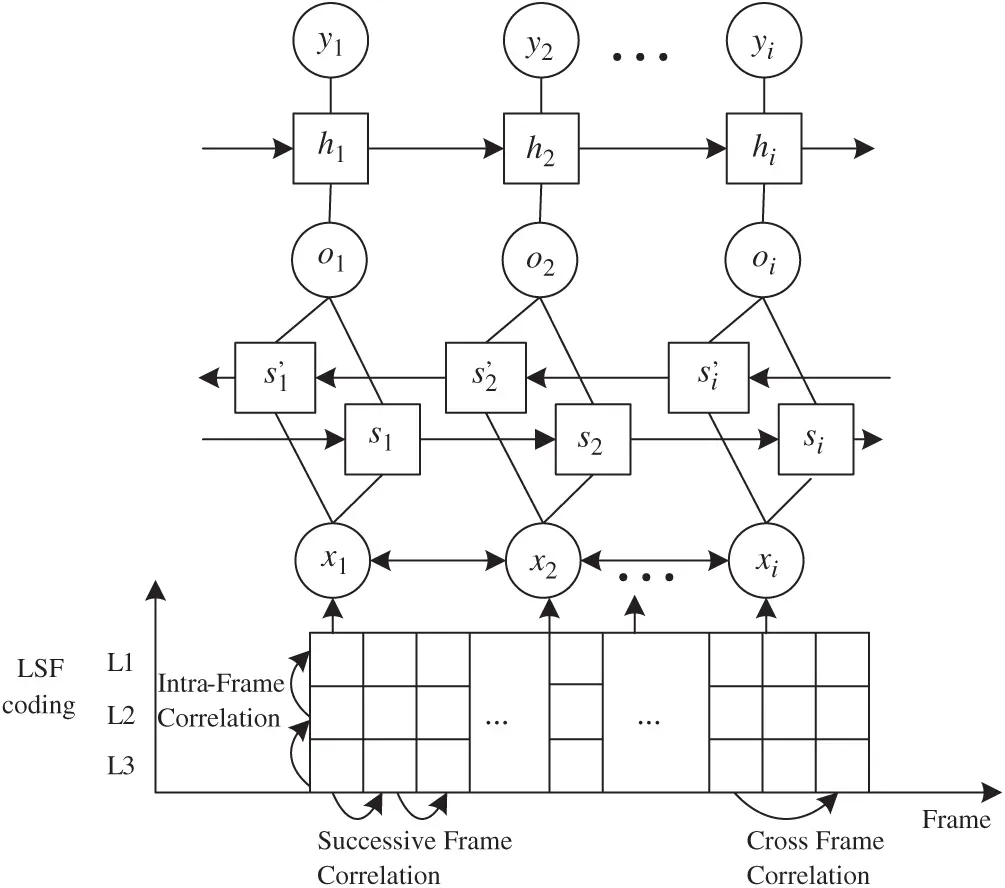Figure 4: Code-word correlation model

Firstly, as Fig. 4, we define all codewords of the T frame in a speech sample as matrix X as follows:

X=[x1,1x1,2x1,Tx2,1x2,2x2,Tx3,1x3,2x3,T](3)

where x1,i, x2,i and x3,i represent the LPC coefficients of frame i in codebooks L1, L2 and L3, respectively. For G.729 encoders, x1,i, x2,i and x3,i are coded with 7 bits, 5 bits and 5 bits, while codebooks L1, L2 and L3 contain 128, 32 and 32 codewords, respectively. Since steganography changes only L1, L2 and L3, X contains all the information used for steganalysis and serves as the input data of the code-word correlation model.

Secondly, we define forward and backward input weights of codewords X to BiLSTM units in the first layer, these weights reflect how much we should value each codeword, which are expressed in a 3×n1 matrix A and A as follows, respectively:

A=[a1,1a1,2a1,n1a2,1a2,2a2,n1a3,1a3,2a3,n1]A=[a1,1a1,2a1,n1a2,1a2,2a2,n1a3,1a3,2a3,n1] (4)

where for each BiLSTM unit u1,i, there are two groups of three input weights a1,i, a2,i and a3,i (a1,i, a2,i and a3,i) multiplied by input codewords x1,i, x2,i and x3,i, respectively, and the two sets of obtained values are added to form the final input value at each time period. To more specific, the input information value for u1,i at time t is

di,t1=(a1,i+a1,i)x1,t+(a2,i+a2,i)x2,t+(a3,i+a3,i)x3,t(5)

Here, let A1,i=a1,i+a1,i, and A2,i and A3,i are obtained in the same way. Then

di,t1=A1,ix1,t+A2,ix2,t+A3,ix3,t(6)

We define D1 as matrix consisting of di,t1 together, i.e.,

D1=[d1,11d1,21d1,T1d2,11d2,21d2,T1dn1,11dn1,21dn1,T1](7)

Then, the output of u1,i at t is:

oi,t1=f(Di,1:t1)=f(ai,1X1,1:t+ai,2X2,1:t+ai,3X3,1:t)(8)

We define O1 as matrix gathering all first layer outputs from start to end when input data is X, i.e.,

O1=[o1,11o1,21o1,T1o2,11o2,21o2,T1on1,11on1,21on1,T1](9)

At each time, each BiLSTM unit can provide independent output based on the input data and the last state to acquire the preliminary past and future information of codewords. To deeply mine the intrinsic relationships of feature data, we stack another LSTM unit layer to the first layer of the BiLSTM unit in this model, as shown in Fig. 4, and O1 is the input data of the second layer network. For simplicity, the second layer of LSTM has only a forward recurrent neural network layer, but the input and output calculation processes of each unit are similar to those of the first layer unit. Therefore, according to the definition and reasoning process from input to output of the BiLSTM unit, the final output matrix Y (as Eq. (10)) given by formula Yj=f(Oj1) of the entire model can be calculated. The matrix Y s also the final correlation feature of all codewords in codebooks L1, L2 and L3, and it can be used for classification.

Y=[y1,1y1,2y1,Ty2,1y2,2y2,Tyn2,1yn2,2yn2,T](10)

3.3 Feature Classification Model

A linear combination of matrix Y can be used as a classification feature to determine whether the original speech is steganographic, but to state the contribution of each feature for classification, the detection weight (DW) matrix E(n2T) of the model output is defined. Before classification, the output matrix Y is multiplied by DW; then, the classification feature O2 is obtained through linear combinations of their products, as given by Eq. (11).

O2=i=1n2j=1TYi,jEi,j(11)

In order to facilitate classification, the output O2 is normalized in [0,1] by the sigmoid function to obtain the final output O3.

O3=S(O2)=S(i=1n2j=1TYi,jEi,j)(12)

We set a detection threshold of 0.5 based on the conventional definition of a threshold in classification. Therefore, the final detection results are determined based on O30.5 (i.e., if O30.5, there is steganography speech; otherwise, it is normal speech).

However, the longer the speech sequence, the faster the growth of the DW matrix, thus slowing the efficiency of training and testing processes. Furthermore, having too many features will increase the possibility of overfitting. To solve this problem, the model is set to output only the feature value of the last unit at T-time; i.e., only y1:n2,T is a classification feature. Note that the final outputs at end time T count on all outputs at all time steps from the first layer because of LSTM's memorizing ability. In addition, DW is reduced to N2 fixed vectors that do not vary with the length of the input sequence. Therefore, the final output features of the correlation model used to classify are calculated as follows:

O3=S(O2)=S(i=1n2Yi,TEi)(13)

3.4 Steganalysis Based on BiLSTM

The steganalysis process of the method is shown in Fig. 5. The specific procedure is as follows:

(1)   The original data is speech samples encoded by G.729a, and the hidden data is obtained by CNV-QIM steganography while encoding. They are segmented by designing sliding windows to acquire more data to train the model.

(2)   The features are extracted from each segment of the original and hidden data to constitute the cover and stego feature sets, which are the codeword sequence of speech coding parameters but not the statistical features of the speech signal, where the stego feature set is positive sample 1 and the cover feature set is negative sample 0.

(3)   Before training the BiLSTM model using features, it is necessary to preprocess the feature sets into the three-dimensional tensor data for input BiLSTM unit, where the first dimension is the number of samples (batch size), the second is dimensionality of samples, and the third is the length of the sample sequence. Next, Multi-layer LSTM cell model is trained with preprocessed feature data. Finally softmax is used to classify the output data of model.

(4)   In the prediction model, the feature data preprocessed in the same way as in Step (3) is input into the trained model for identification, then the model outputs a two-dimensional matrix as a result. The first dimension of the matrix is the number of feature samples, and the second dimension is the mark of seganogrphy or not.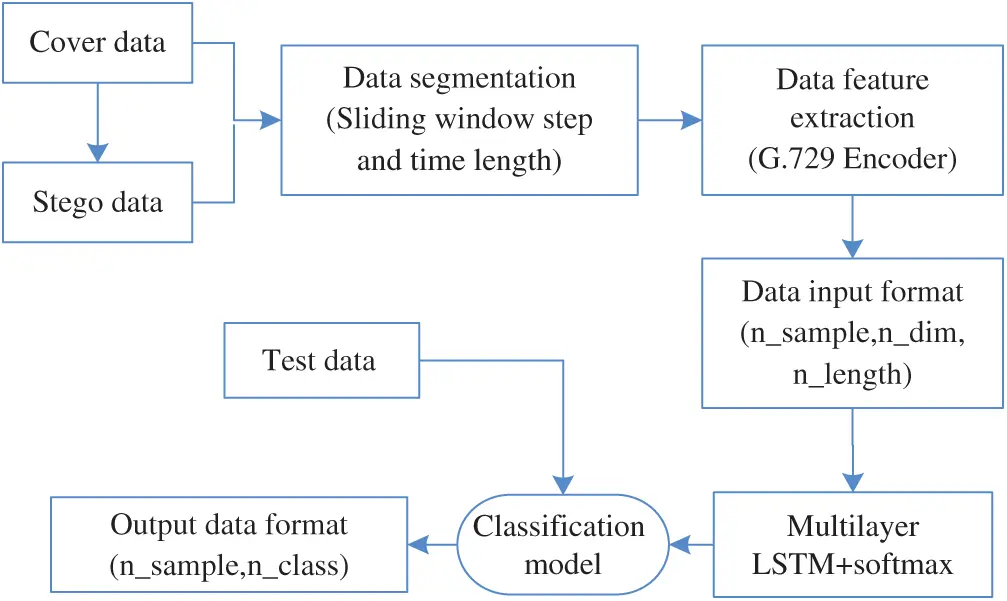Figure 5: Steganalysis flow chart

4  Experiment and Result Analysis

For an excellent CNV-QIM steganography algorithm based on codebook index in LPC domain, we design steganalysis algorithm based on BiLSTM network and sliding window and carry out experiments (take G.729a coder as an example). The experiment is mainly divided into two processes: the first is the collection and preprocessing of the speech corpus, the second is the training of the model and the comparative experiment.

4.1 The Collection and Preprocessing of Corpus

(1)   We collect 98 h of Chinese and English speech samples which are from different male and female speakers in PCM format for forming hybrid speech sample dataset. And these speech samples are filtered through a second-order high-pass filter with a cut-off frequency of 140 Hz.

(2)   Filtered speech is encoded by G.729A and segmented into 3, 5 and 10 s samples, respectively, by the sliding window method to form the original speech sample.

(3)   The original speech is framed by 80 sample points (10 ms) for short-term LPC analysis. The obtained LPC coefficient is converted to an LSF coefficient for 2-level vector quantization. The first level is a 10-dimensional codebook coded with 7 bits, at the second level of quantification, the 10-dimensional vector is split into two 5-dimensional vectors coded with each 5 bits. The corresponding codebooks are L1, L2 and L3, respectively, and the codewords are extracted from them to form the cover features.

(4)   The CNV steganography is performed while encoding the samples in Step (2), and 7 bits, 5 bits and 5 bits codewords in three codebook L1, L2 and L3 are modified in steganography. Thus, only these codewords are extracted for detection to constitute stego features.

(5)   In order to verify relationship among embedding rate, sample duration and detection accuracy, the embedding rates of samples in this experiment are 100%, 50% and 20%, respectively, and the sample length of each embedding rate is segmented into 3, 5 and 10 s.

4.2 The Training of the Model and Comparative Experiment

The preprocessed original and steganographic features of samples are mixed. For example, the original feature of 3 s and the steganographic feature of 3 s with 100% embedding rate are blended. Next, 70% of the mixed features (cover and stego) are applied to train the BiLSTM model, and the remaining 30% are used to evaluate the model. Finally, 9000 mixed samples are randomly selected to test the model. Considering the detection accuracy, training time and testing time, we set two layers of LSTM in training model, each of which has 50 units; dropout_W = 0.2, dropout_U = 0.2, loss = ‘binary_crossentropy’, optimizer = ‘adam’, and batch_size = 32. We use classification accuracy rate, false positive rate (FP) and false negative rate (FN) to evaluate the performance of model. In the model constructed in this paper, notably, the number of BiLSTM units N1 and LSTM units N2 is particularly important. Therefore, the accuracy, training time and prediction time of the four models with N1 = 40, N2 = 50, N1 = 50, N2 = 60, N1 = 60, N2 = 50, and N1 = N2 = 50 are designed and firstly compared in the experiment, the results are shown in Tab. 1. As can be seen from Tab. 1, the accuracy of the model with N1 = N2 = 50 is higher than the other two models, and its training time and prediction time are the shortest. In general, the model with N1 = N2 = 50 is selected for the subsequent comparative experiments, namely, the method in this paper is compared with the detection methods of RNN-SM , IDC (index distribution characteristics)  and SS-QCCN . In addition, IDC and SS-QCCN are realized based on SVM, and they cannot detect large samples effectively. Therefore, we randomly extract 4000 samples from mixed features for training IDC and SS-QCCN model and 2000 samples for testing their models. In particular, in order to show the advantages of our detection method for low embedding steganography in short-term speech, the comparative experiments of four methods when the embedding rate of 3%, 5% and 9%, durations of 3 s are conducted. The experimental results of various methods are shown in Tabs. 24.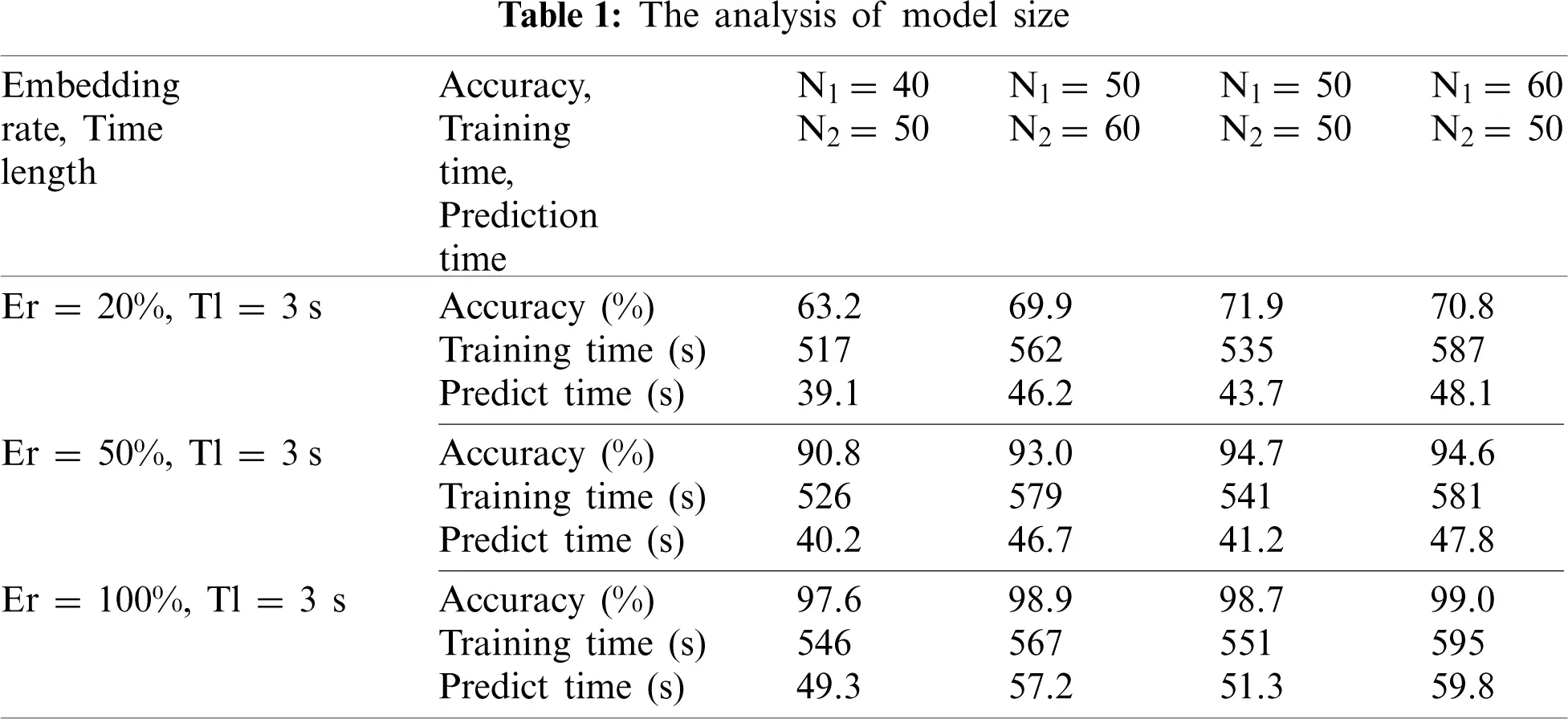It can be seen from Tabs. 24 that the detection accuracy of four methods increases with the raising of time length and embedding rate. When the embedding rate is greater than 50% and various durations, the detection accuracy of four methods is almost above 90%. and SS-QCCN has a slight advantage, because it selects a large number of high-dimensional features for detection. However, No matter what length of time, when the embedding rate is less than 50%, the accuracy of our method in this paper is obvious superior than that of the other methods. Especially when the embedding rate is 20% and the time length is 10 s, the detection accuracy of the proposed method reaches 88.8%, which is the only detection result close to 90% among the four methods. It can be explained that the proposed method is more suitable for steganography detection in short-term speech with low embedding rate, which is one of the main purposes of this experiment. In order to further verify advantages of the proposed method in steganography detection for low embedding speech, we add detection experiments of four methods with embedding rate of 3%, 5% and 9% and time length is 3 s, the results are shown in Tab. 2. From Tab. 2 that the detection effect of method in this paper is the best of the four methods, when the embedding rate is less than 10% and the same time length. Especially when the embedding rate is only 9%, the detection accuracy of our method reaches 60.7% and shows great advantages over other models (Ours (60.7%) vs. RNN-SM (51.9%)), existing models represent unsatisfactory detection performance and their detection results are less than 50%. The reason may be that the input layer of our model can fully represent the correlation features of inter-frame and intra-frame codeword while transforming features from low dimension to high dimension. Generally speaking, the detection result of IDC is relatively unsatisfactory among the four methods, when the embedding rate is less than 50%. The possible reason is that it uses SVM method, which is suitable for small sample classification, and its feature dimension is low.In order to express the relationship between detection accuracy and embedding rate and time length intuitively, ROC curves at embedding rates of 20% and 50%, durations of 3 s are shown in Figs. 6a and 6b. At the same time, the detection accuracy of proposed method is compared with the other three methods at the embedding rate of 20% and 50%, durations of 3, 5 and 10 s, as illustrated in Fig. 7. It can be clearly seen from the diagram that the consequence is consistent with the result of analysis for table data. In addition, to enable online steganalysis, the time for testing each sample should be as short as possible to improve the detection efficiency. Therefore, the average detecting time of proposed method is compared with the other three methods when the embedding rate is 100% in each time length, and the results are shown in Tab. 5.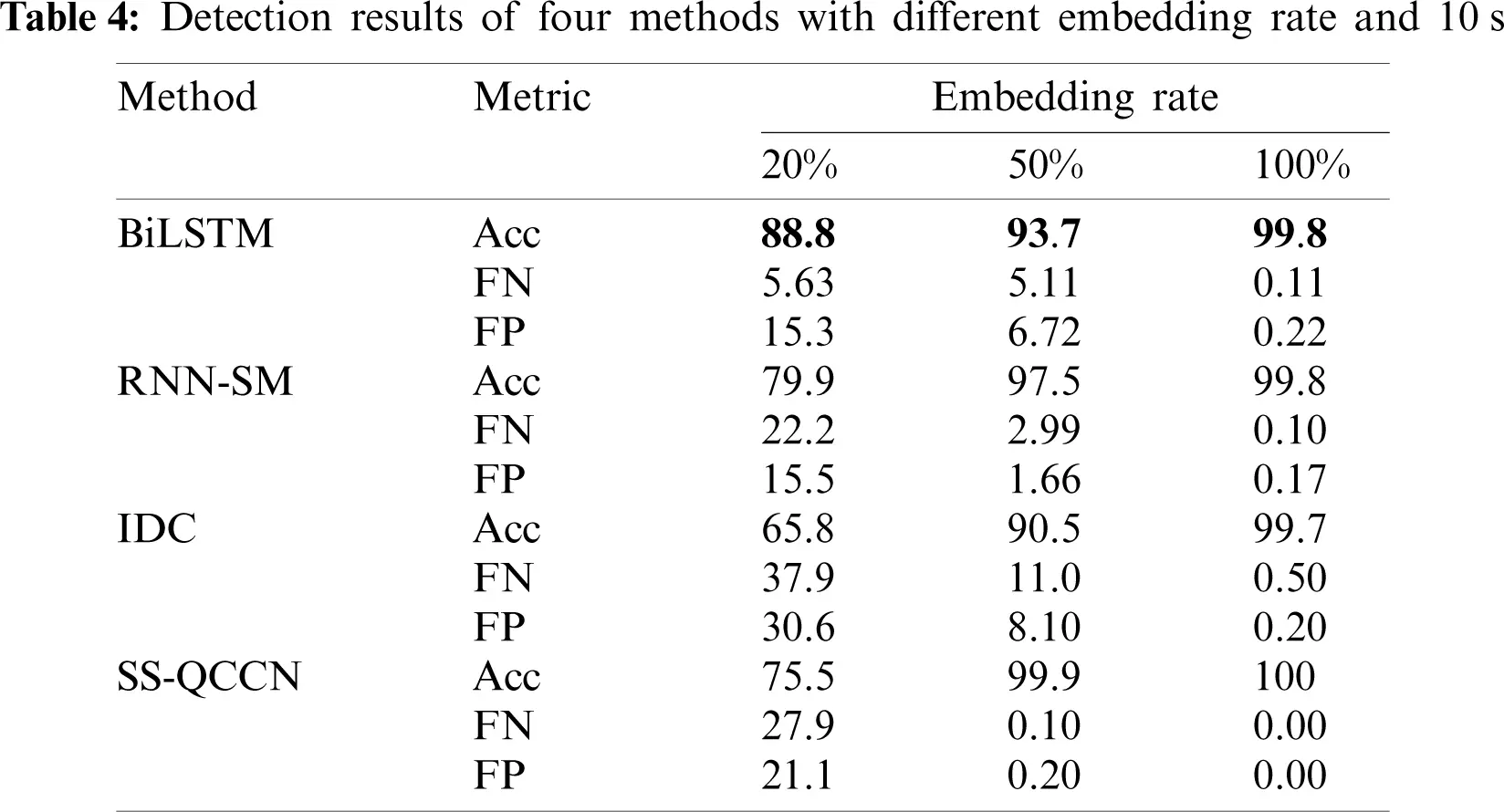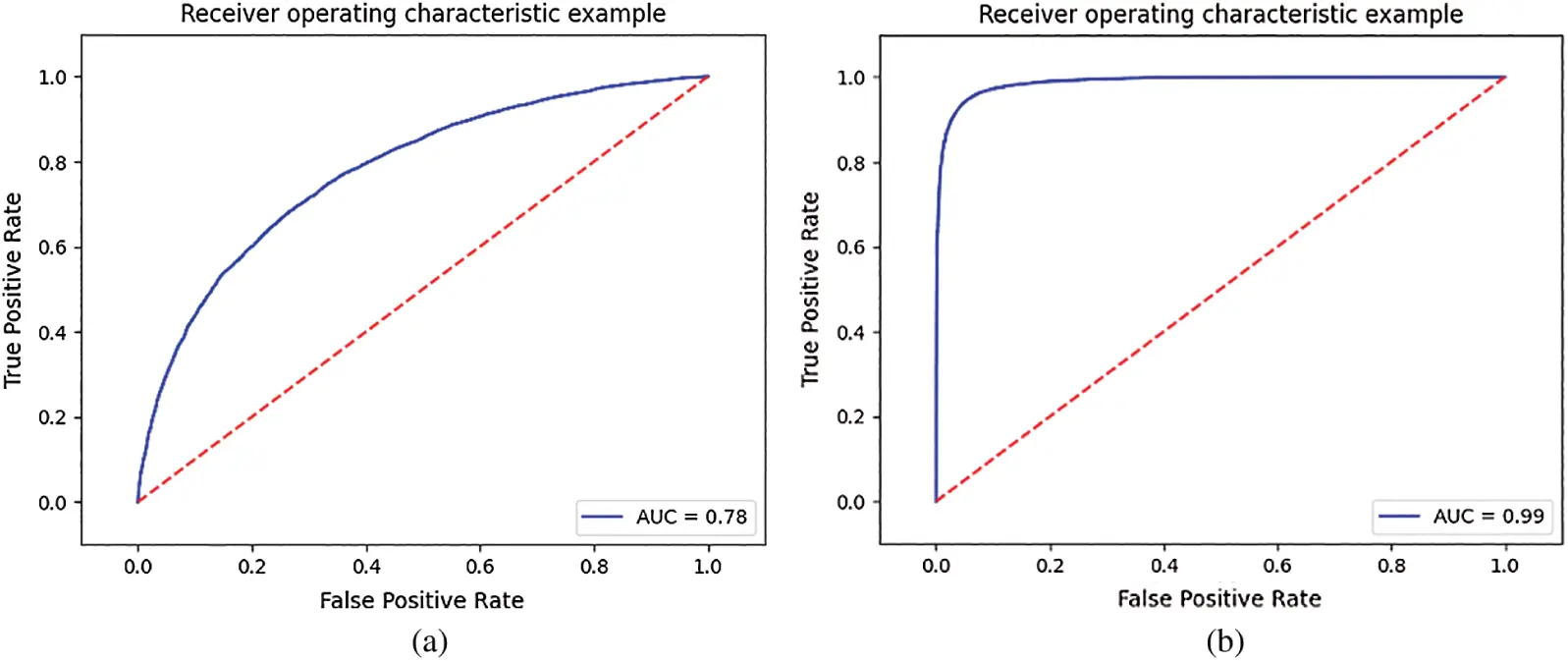Figure 6: ROC curves at different embedding rates and durations of 3 s (a) 20%, 3 s (b) 50%, 3 s

It can be found out from Tab. 5 that although the number of BiLSTM units in this model is more than that of RNN-SM, the average testing time of the model is second only to that of RNN-SM model, and testing time of both models is less than 10% of the sample time length, which can realize real-time steganography detection. However, the overhead of SS-QCCN is distinctly higher than the other three methods due to compute a high dimensional feature vector and need to perform PCA reduction. Combined with the detection accuracy and testing time, the method proposed in this paper is obviously better than other methods.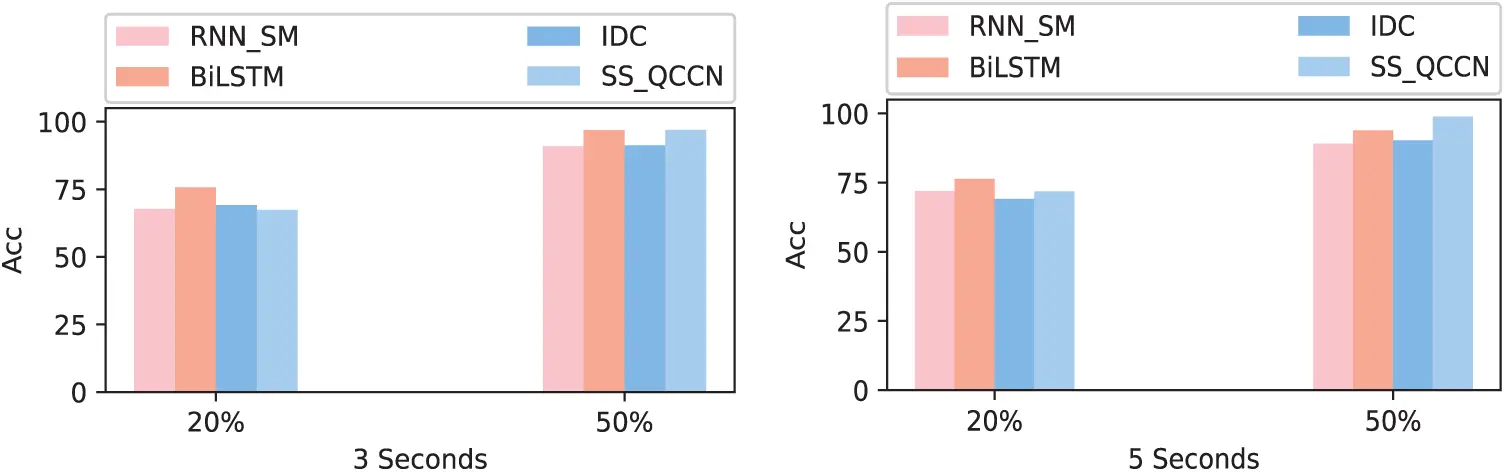Figure 7: Accuracy comparison of four models with embedding rate of 20% and 50% at duration 3, and 5 s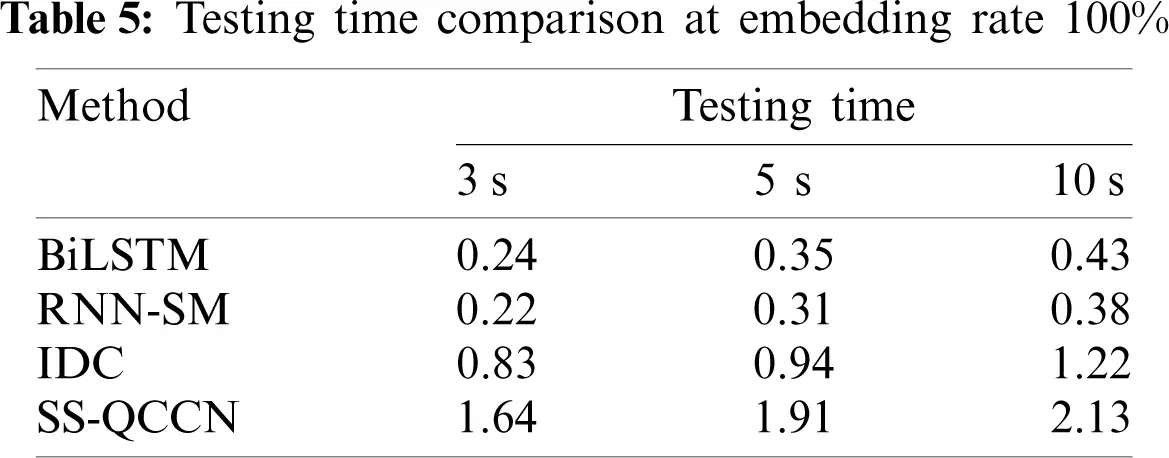5  Conclusion and Innovation

To detect CNV-QIM steganography with low embedding rate in low bit-rate speech codec, a steganalysis algorithm based on the BiLSTM neural network model is proposed in this paper, and it achieved good detection results for CNV-QIM steganography of low bit-rate speech codec in VoIP streams. Compared with RNN-SM, IDC and SS-QCCN, the method in this paper has a higher detection accuracy for short samples with low embedding rates. When the embedding rate is 20% and the duration is 3 s, the detection accuracy reaches 75.7%, which is higher than that of the RNN-SM method by up to 11.7%. Especially when the embedding rate is only 9% and durations of 3 s, the detection accuracy of our method reaches 60.7% and shows great advantages over other models. Furthermore, in terms of detection efficiency, the average testing time of samples (100% embedding) is 0.3 s, which shows that the method can realize real-time steganography detection of VoIP streams.

There are two innovations in this paper: (1) Accurate steganography detection in low bit rate speech with low embedding rate is realized by building code-word correlation model using BiLSTM. (2) Real-time detection for timely voice is achieved.

Acknowledgement: This work was supported by grants from the National Natural Science Foundation of China (No. 61862002). The authors would like to thank the anonymous reviewers for their valuable suggestions.

Funding Statement: This research was supported by the National Natural Science Foundation of China (No. 61862002).

Conflicts of Interest: The authors declare that they have no conflicts of interest to report regarding the present study.

## References

1. Tian, H., Sun, J., Chang, C. C., Qin, J., & Chen, Y. (2017). Hiding information into voice-over-ip streams using adaptive bitrate modulation. IEEE Communications Letters, 21(4), 749-752. [Google Scholar] [CrossRef]
2. Qin, C., & Hu, Y. C. (2016). Reversible data hiding in vq index table with lossless coding and adaptive switching mechanism. Signal Process, 29, 48-55. [Google Scholar] [CrossRef]
3. Huang, Y. F., Liu, C. H., & Tang, S. Y. (2012). Steganography integration into a low-bit rate speech codec. IEEE Transactions on Information Forensics and Security, 7(6), 1865-1875. [Google Scholar] [CrossRef]
4. Tian, H., Sun, J., Chang, C. C., Huang, Y., & Chen, Y. (2018). Detecting bitrate modulation-based covert voice-over-ip communication. IEEE Communications Letters, 22(6), 1196-1199. [Google Scholar] [CrossRef]
5. Xiao, B., Huang, Y. F., Tang, S. Y. (2008). An approach to information hiding in low bit-rate speech stream. IEEE Global Telecommunications Conference, pp. 1–5. New Orleans, LA, USA.
6. Yan, D. Q., & Wang, R. D. (2014). Detection of m-p3stego exploiting recompression calibration-based feature. Multimedia Tools and Applications, 72(1), 865-878. [Google Scholar] [CrossRef]
7. Guo, H., Yan, D., Wang, R., Wang, Z., & Wang, L. (2015). Mp3 steganalysis based on difference statistics. Computer Engineering and Applications, 51(7), 88-92. [Google Scholar]
8. Tian, H., Wu, Y., Huang, Y., Liu, J., Chen, Y. et al. (2015). Steganalysis of low bit-rate speech based on statistic characteristics of pulse positions. 10th International Conference on Availability, Reliability and Security, pp. 455–460. Toulouse, France, August.
9. Li, S. B., Jia, Y. Z., Fu, J. Y., & Dai, Q. X. (2014). Detection of pitch modulation information hiding based on code-book correlation network. Chinese Journal of Computers, 37(10), 2107-2116. [Google Scholar]
10. Li, S. B., Huang, Y. F., & Lu, J. C. (2013). Detection of QIM steganography in low bit-rate speech codec based on statistical models and SVM. Chinese Journal of Computers, 36(6), 1168-1176. [Google Scholar] [CrossRef]
11. Li, S. B., Jia, Y. Z., & Kuo, C. C. (2017). Steganalysis of QIM steganography in low-bit-rate speech signals. IEEE/ACM Transactions on Audio, Speech, and Language Processing, 25(5), 1011-1022. [Google Scholar] [CrossRef]
12. Li, S. B., Tao, H. Z., & Huang, Y. F. (2012). Detection of quantization index modulation steganography in G. 723.1 bit stream based on quantization index sequence analysis. Journal of Zhejiang University-SCIENCE C, 13(8), 624-634. [Google Scholar] [CrossRef]
13. Yang, Z. L., Guo, X. Q., Chen, Z. M., Huang, Y. F., & Zhang, Y. J. (2018). RNN-stega: Linguistic steganography based on recurrent neural networks. IEEE Transactions on Information Forensics and Security, 14(5), 1280-1295. [Google Scholar] [CrossRef]
14. Yang, Z. L., Zhang, S. Y., Hu, Y. T., Hu, Z. W., & Huang, Y. F. (2020). VAE-Stega: Linguistic steganography based on variational auto-encoder. IEEE Transactions on Information Forensics and Security, 16, 880-895. [Google Scholar] [CrossRef]
15. Graves, A., & Schmidhuber, J. (2005). Framewise phoneme classification with bidirectional lstm and other neural network architectures. Neural Networks, 18(5), 602-610. [Google Scholar] [CrossRef]
16. Chen, C. T., Zhuo, R., & Ren, J. T. (2019). Gated recurrent neural network with sentimental relations for sentiment classification. Information Sciences, 502, 268-278. [Google Scholar] [CrossRef]
17. Jorge, C. Z., Alejandro, H. T., & Enrique, V. (2019). Handwritten music recognition for mensural notation with convolutional recurrent neural networks. Pattern Recognition Letters, 128, 115-121. [Google Scholar] [CrossRef]
18. Lin, Z. N., & Huang, Y. F. (2018). Rnn-sm: Fast steganalysis of voip streams using recurrent neural network. IEEE Transactions on Information Forensics and Security, 13(7), 1854-1868. [Google Scholar] [CrossRef]
19. Yang, Z., Huang, Y., Jiang, Y., Sun, Y., & Zhang, Y. J. (2018). Clinical assistant diagnosis for electronic medical record based on convolutional neural network. Scientific Reports, 8(1), 6329. [Google Scholar] [CrossRef]
20. Srivastava, N., Hinton, G., Krizhevsky, A., Sutskever, I., & Salakhutdinov, R. (2014). Dropout: A simple way to prevent neural networks from overfitting. Journal of Machine Learning Research, 15(1), 1929-1958. [Google Scholar]This work is licensed under a Creative Commons Attribution 4.0 International License, which permits unrestricted use, distribution, and reproduction in any medium, provided the original work is properly cited.